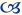# What is a “Discount Rate”?

This article first appeared in the spring 1996 issue of the Expert Witness.

Alberta is one of only four provinces in which the discount rate is not mandated. As I argued in Ontario’s 2 1/2% Solution (Canadian Bar Review, December 1982) this means that we are able to react much more flexibly to changes in the economic situation than are the six provinces whose rates are set by a central authority. Indeed, the superiority of Alberta’s approach is seen in the fact that whereas interest rates have varied significantly over the last 15 years, not one of the provinces with mandated rates has adjusted that rate.

Nevertheless, the lack of a mandated rate does carry the drawback that an onus is placed both on financial expert witnesses and on counsel to understand how the discount rate is determined and to identify whether economic forces have changed in such a way as to make previous assumptions about that rate obsolete. The purpose of this article will be to provide a basic explanation of what the discount rate is and of how it works. In a second article, I identify a number of alternative methods of forecasting the discount rate and use what is generally considered to be the preferred method to identify such a rate for Alberta.

Assume that a plaintiff will require dental work one year from now. If that work was carried out today, it would cost \$1,040. The question which faces the legal system is: “How much does the plaintiff have to be compensated today, in order to ensure that he/she will have enough money to pay for this procedure one year from now?” The answer to this question depends, first, upon the effect of the rate of inflation on the cost of the procedure; and, second, upon the rate of interest at which the plaintiff can invest his/her award.

The effect of the rate of inflation is relatively straight forward. If, for example, the cost of dental procedures is expected to increase by 2.5 percent in the next year, this plaintiff will need \$1,040 increased by 2.5 percent one year from now. That is, the amount required will be:

\$1,040 + (2.5% x \$1,040)
= (1.00 x \$1,040) + (0.025 x \$1,040)
= (1.00 + 0.025) x \$1,040
= 1.025 x \$1,040
= \$1,066

In short, to find the inflated value one year from now, the current value (here, \$1,040) is multiplied by 1 plus the rate of inflation (here, 1.025).

The second step is to determine how much has to be paid to the plaintiff today in order to ensure that he /she will have \$1,066 one year from now. Assume for this purpose that the rate of interest which plaintiffs can expect to receive on secure investments is 6.6 percent per annum. It is intuitively clear that \$1,000 invested at this rate will yield \$1,066 (the desired amount) one year from now. Formally, this \$1,000 figure, which is called the present discounted value or commuted value of \$1,066, can be derived in the following way: Call the present discounted value \$P. When \$P is invested at 6.6 percent interest, we want it to yield \$1,066. Hence,

\$P + (6.6% x \$P) = (1.00 x \$P) + (0.066 x \$P)
= (1.00 + 0.066) x \$P
= 1.066 x \$P
= \$1,066

That is, we know that

1.066 x \$P = \$1,066

Therefore, to find \$P, we need only divide both sides of this equation by 1.066, to obtain

\$P = (\$1,066 / 1.066)
= \$1,000

Remembering that the \$1,066 figure in this equation was found by increasing the current cost of the dental procedure, \$1,040, by the rate of inflation, 2.5 percent, it is now seen that amount which must be paid to the plaintiff today, \$P, may be obtained from the formula:

\$P = \$1040 x (1.025 / 1.066)
= \$1,040 x (1.00 / 1.04)
= \$1,000

What this set of calculations is intended to show is, first, that \$P can be found by multiplying the current cost of the expense to be compensated, here \$1,040, by (1 + inflation), here 1.025, divided by (1 + interest), here 1.066. Second, (1.025 divided by 1.066) can be replaced by (1.00 divided by 1.04). This 1.04 figure is known by economists as the real rate of interest or the discount rate. This is the figure which expert witnesses use to determine the present, or lump sum value of a future cost. It is called the real rate of interest because it was calculated by dividing 1.066 by 1.025; that is, (1.025/1.066) = 1.00/(1.066/1.025) = (1.00/1.04). Dividing (1 + interest) by (1 + inflation) in this way has the effect of “netting out” the impact of inflation from the observed, or nominal, interest rate, leaving only that element of interest payments which is independent of inflation – the “real” rate of interest.

Economists and other financial experts have used the real rate of interest to discount future losses because it has been less volatile than the nominal rate of interest. (The nominal rate increases and decreases with the rate of inflation while the underlying real rate remains stable.) Recently, however, the real rate has been almost as variable as the nominal rate. Nevertheless, because the courts have become accustomed to the use of the real rate, the Expert Witness will follow that convention.Christopher Bruce is the President of Economica and a Professor of Economics at the University of Calgary. He is also the author of Assessment of Personal Injury Damages (Butterworths, 2004).